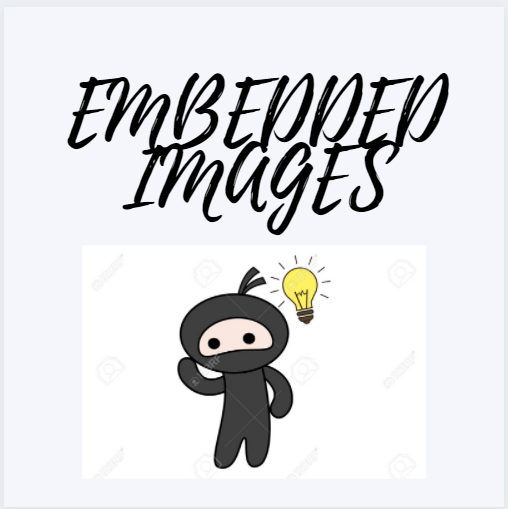# Embedded Images

## Introduction:

Embedded images are images one inside the other figure. Embedded figure questions consist of a unique figure which is hidden or embedded in one of the four option figures. In such questions, all the options look the same and are confusing. So candidates need to be careful while attempting such questions. Anyhow with practice, we can master these embedded figures. We must basically understand the structure of the given question figure and then proceed to find the correct answer figure.

Embedded questions are one of the most important questions during the aptitude test for any company. From the statistics embedded questions 5-10% of the question paper.## Type of Embedded Images problems:

The embedded Images test is to assess how quickly a candidate can recognize a figure that is hidden among other figures. In each question, there is a model figure and four answer figures. The candidate has to look for the model from the answer figures.

### Type 1 Complex Answer Figure:

A question figure (x) is given followed by four complex option figures in such a way that the question figure (x) is embedded in only one of the given four options. The candidate must identify the correct figure in which figure (x) is hidden.

Example :

Here we have a figure named X. The task is to find X in the other four images. So which of the four images contains the figure X?

Solution:

In the above example, we see that figure X is not present in (2), or (4). Also (3) seems to contain X but as you look at the bottom, you will find out that even (3) is not the right answer. So that leaves (1). if you were to take X and put it in (1), it will not make any difference to either of the two figures. So the correct answer here is (1). You will need to take into account the orientation too. This means that in case you don’t find X to fit a particular option, you will have to change the orientation of one of the two figures. In other words, we say that X is embedded in (1). You can see that in the below figure:

Example :

Can you find the figure out of the following group, that contains the figure X?

Solution:

You may be tempted to choose (2) or (4) but that would be wrong. If you look at option (2), you will see that the orientation is not correct. Also in (4) even though the orientation is correct, the figure is split at points and thus not the correct option. Also, you will easily see that (1) is not the correct option as it neither has the orientation and nor the symmetry. This leaves the option (3). Figure X can be plotted on (3) without changing either of the two figures. This can be seen as below:

### Type 2 Complex Question Figure:

A complex question figure (x) is given followed by four option figures in such a way that only one of the option figures is embedded inside the given question figure. The candidate must identify the correct option figure which is hidden inside the question figure (x).

Example :

In the given question a complex figure is given. Find out which of the simple figure given in the alternatives is hidden in the complex figure?

Solution:

Option B

From the figure, the intersection part of right-most and bottom circles contains two curve lines inside the intersection part which looks the same as it is given in figure B, and also the part of the bottom figure which is extended towards the right.

Example :

From the following figures, choose the odd one out!

A) 1             B) 2              C) 3                 D) 4                E) 5           F) None of these

Solution:

All the images contain the same star at the center. The color and even the shade are the same. So there is no point in wasting time on it. The difference lies in the number of stars and their arrangements around the central star. Again we see that the number of smaller stars is different for almost all the figures except (2) and (5) which both have one star each. As a result, we can say that it is not the number of these little stars that determines whether a given figure belongs or not. So it must be the orientation.

1. What is an embedded image?

Embedded images are images that can be integrated directly into the email source code. Embedded images do not need to be downloaded by the recipient; they are shown directly in the email program.

2. What is an embedded image in reasoning?

Embedded Images test is to assess how quickly can a candidate recognize a figure that is hidden among other figures. In each question, there is a model figure and four answer figures. The candidate has to look for the model from the answer figures.

3. What is meant by embedded figures?

Embedded figures are the figures hidden inside the other figure. In other words, a figure (X) is said to be embedded inside figure (Y), if figure (Y) contains a part of Figure (X).

## Key Takeaways:

In this blog, we mentioned all different types of embedded images, followed by some examples and approaches to solve them. Practicing embedded images questions will help you to clear your first round. Embedded images make 5-10% of the question paper during your aptitude exam.

Practice more and do your best..!!

Happy Learning!!

By Dharani Mandla